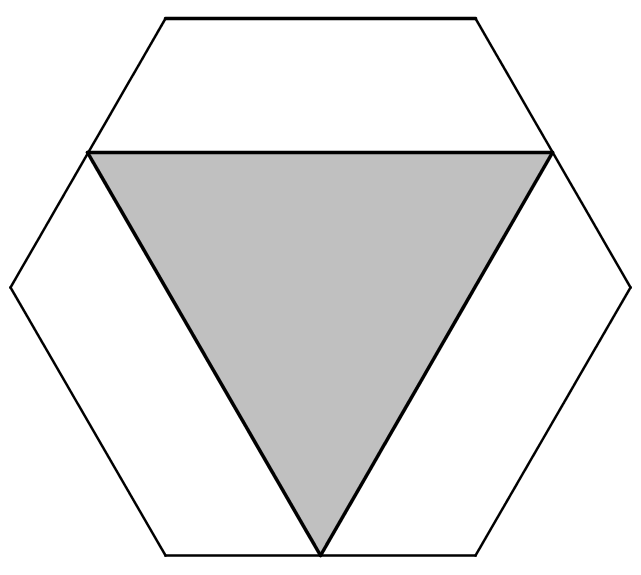You may also likeIsosceles

Prove that a triangle with sides of length 5, 5 and 6 has the same area as a triangle with sides of length 5, 5 and 8. Find other pairs of non-congruent isosceles triangles which have equal areas.Four rods, two of length a and two of length b, are linked to form a kite. The linkage is moveable so that the angles change. What is the maximum area of the kite?Estimating Angles

How good are you at estimating angles?

Perimeter in a Hexagon

Age 11 to 14 ShortChallenge LevelThe diagram shows a regular hexagon with sides of length 14 cm.

An equilateral triangle has been inscribed so that the vertices of the triangle are midpoints of the sides of the hexagon.

What is the perimeter of the triangle?

This problem is taken from the UKMT Mathematical Challenges.

You can find more short problems, arranged by curriculum topic, in our short problems collection.